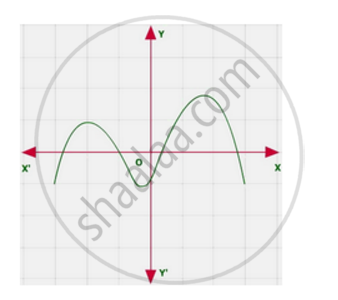# The Graphs of Y = P(X) Are Given in Following Figure, for Some Polynomials P(X). Find the Number of Zeroes of P(X) - Mathematics

The graphs of y = p(x) are given in following figure, for some polynomials p(x). Find the number of zeroes of p(x)#### Solution

The graph intersects x-axis at four point.

∴ The no of zeroes of p(x) is four

Concept: Geometrical Meaning of the Zeroes of a Polynomial
Is there an error in this question or solution?
Chapter 2: Polynomials - Exercise 2.1 [Page 28]

#### APPEARS IN

NCERT Class 10 Maths
Chapter 2 Polynomials
Exercise 2.1 | Q 1.5 | Page 28
Share